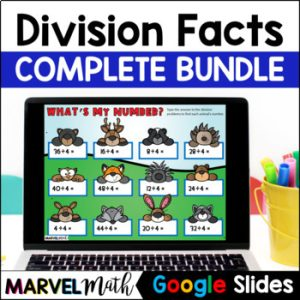Save 10% on your first order with code EXTRA10

# Division Facts ÷ 11 FUN Digital Activities

\$3.00\$19.99
SKU: 5529979 Categories: ,

## Description

The FUN and ENGAGING Division Practice Activities are Perfect for Distance Learning. Students always need to practice their math facts, and they will enjoy these Simple and Easy-to-Use Google Slides.

⭐ Watch the Video Preview

This set includes all of the ÷ 10 math facts. All Facts 11 ÷ 11 = 1 through 132 ÷ 11 = 12 are included in this resource.

Topics Include:

• Math Facts practice with a mix of movable pieces and places to type in answers to show true memorization
• Related fact activities to help students bridge from multiplication to division
• Input-Output Tables and Number Machines

What’s Included?

• 2 Sets of Digital Flashcards for students to use again and again
• 10 Interactive Activities
• Teacher Key
• Directions on how to assign the Google Slides activity to students in Google Classroom™

Standards Alignment

CCSS.3.OA.C.7 Fluently multiply and divide within 100, using strategies such as the relationship between multiplication and division (e.g. knowing that 8 x 5 = 40, one knows 40 ÷ 5 = 8) or properties of operations. By the end of Grade 3, know from memory all products of two one-digit numbers.

CCSS.3.OA.B.6 Understand division as an unknown-factor problem. For example, find 32 ÷ 8 by finding the number that makes 32 when multiplied by 8.

TEKS 3.4F Recall facts to multiply up to 10 by 10 with automaticity and recall the corresponding division facts.

TEKS 3.5D Determine the unknown whole number in a multiplication or division equation relating three whole numbers when the unknown is either a missing factor or product.